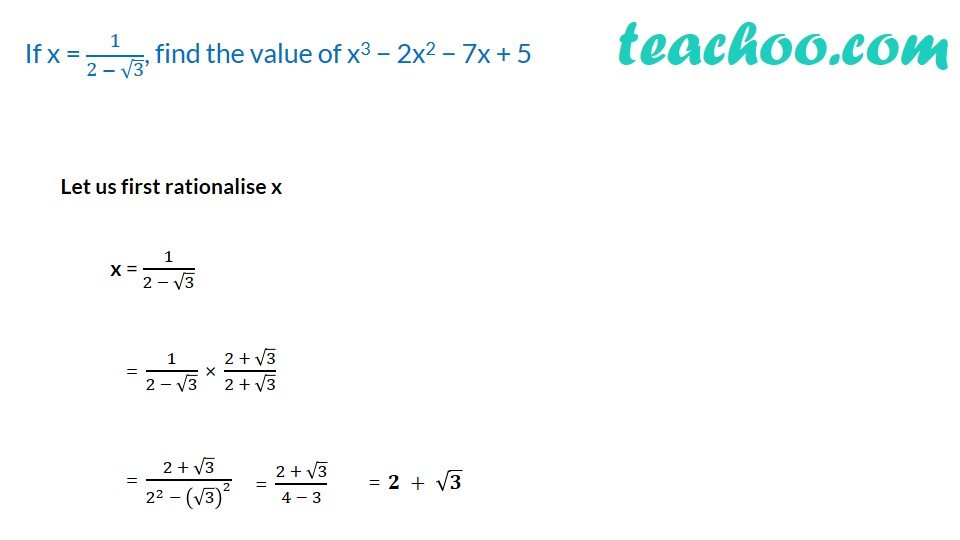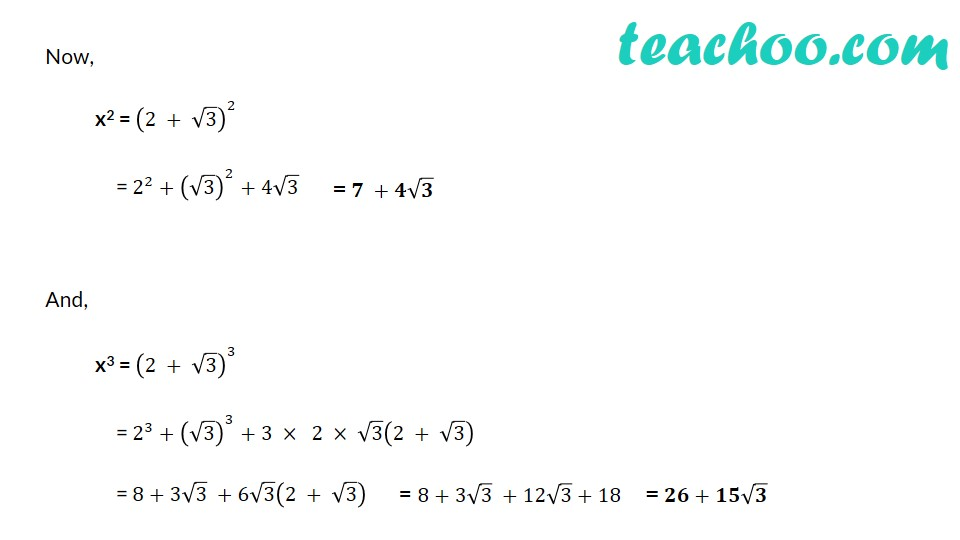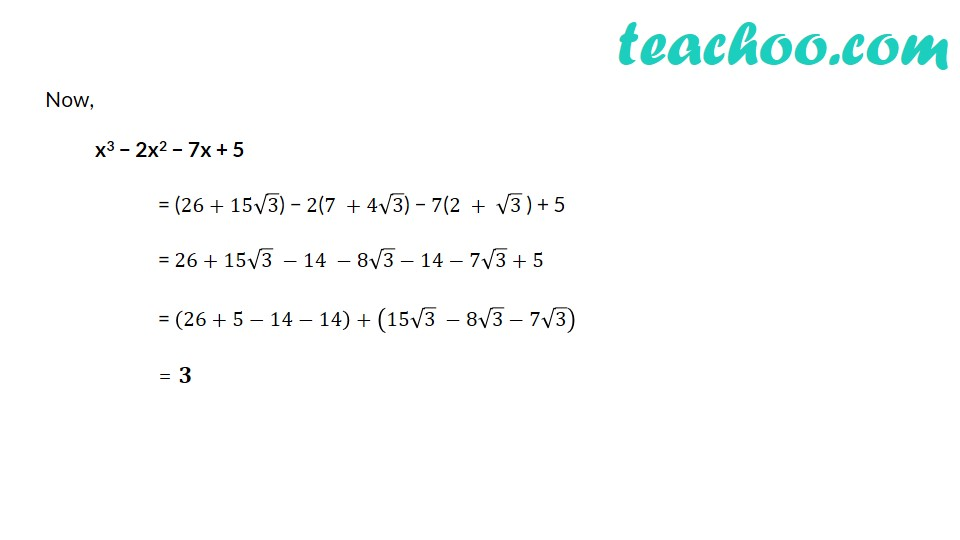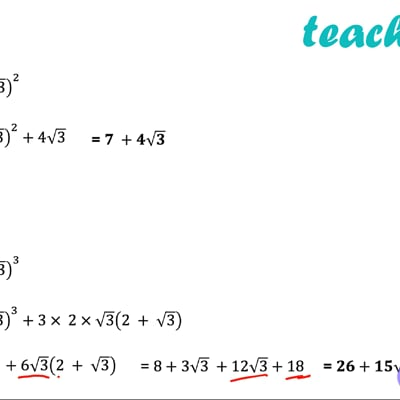Rationalising

Chapter 1 Class 9 Number Systems
Concept wiseThis video is only available for Teachoo black users

Introducing your new favourite teacher - Teachoo Black, at only ₹83 per month

### Transcript

If x = 1/(2 − √3), find the value of x3 − 2x2 − 7x + 5 Let us first rationalise x x = 1/(2 − √3) = 1/(2 − √3) × (2 + √3)/(2 + √3) = (2 + √3)/(2^2 − (√3)^2 ) = (2 + √3)/(4 − 3) Now, x2 = (2 + √3)^2 = 〖2^2+(√3)〗^2 + 4√3 And, x3 = (2 + √3)^3 = 〖2^3+(√3)〗^3 + 3 × 2 × √3 (2 + √3) = 8+3√3 +6√3 (2 + √3) = 8+3√3 +12√3+18 Now, x3 − 2x2 − 7x + 5 = (26+15√3) − 2(7 +4√3) − 7(2 + √3 ) + 5 = 26+15√3 −14 −8√3−14−7√3+5 = (26+5−14−14)+(15√3 −8√3−7√3) = 𝟑